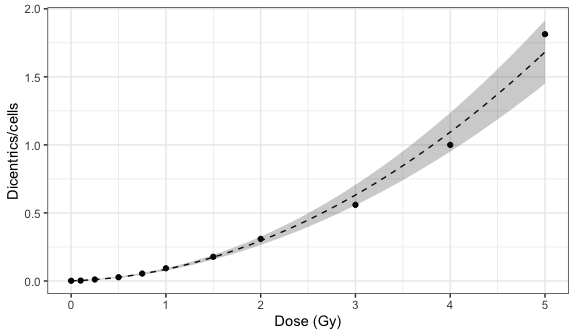# Dicentrics dose-effect fitting

## Input count data

The first step is to input the count data. On the app, we can select to either load the count data from a file (supported formats are .csv, .dat, and .txt) or to input the data manually. Once the table is generated and filled, the “Calculate parameters” button will calculate the number of cells ($$N$$), number of aberrations ($$X$$), as well as mean ($$\bar{y}$$), variance ($$\hat{\sigma}^{2}$$), dispersion index ($$\hat{\sigma}^{2}/\bar{y}$$), and $$u$$-value.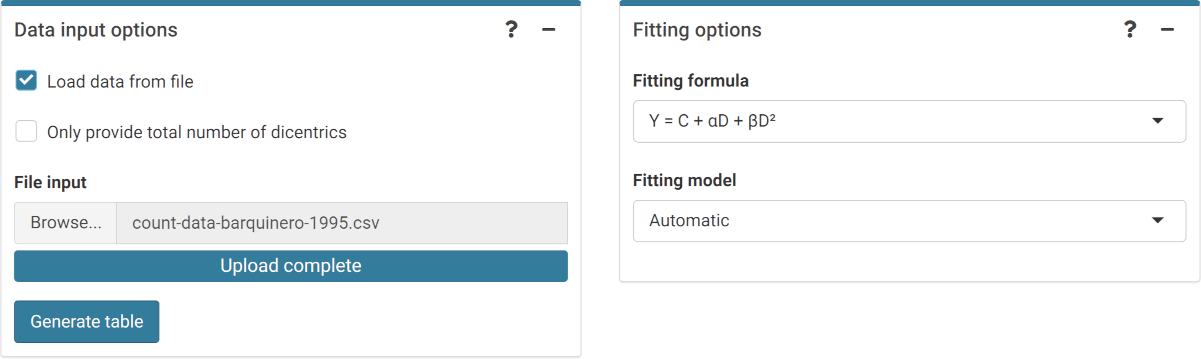‘Data input options’ and ‘Fitting options’ boxes in the dose-effect fitting module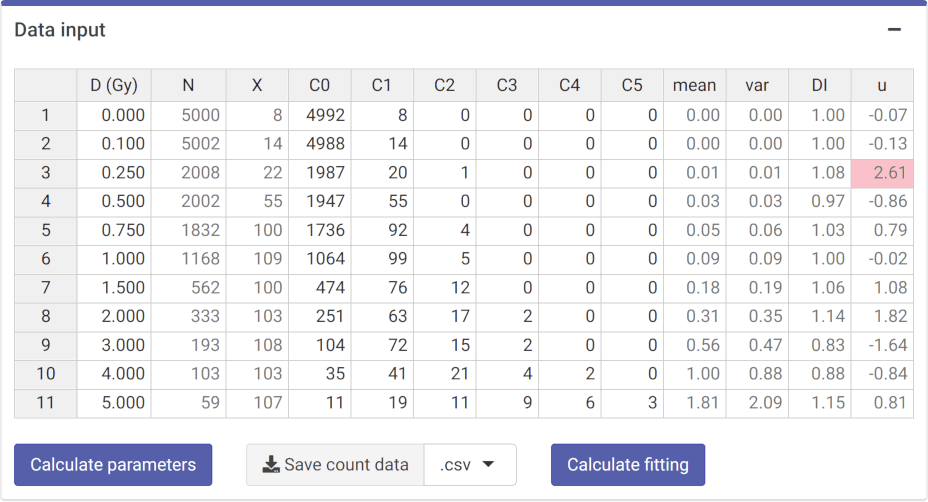‘Data input’ box in the dose-effect fitting module

This step is accomplished in R by calling the calculate_aberr_table() function:

count_data <- system.file("extdata", "count-data-barquinero-1995.csv",
package = "biodosetools") %>%
calculate_aberr_table(type = "count")
count_data
#> # A tibble: 11 × 13
#>        D     N     X    C0    C1    C2    C3    C4    C5    mean     var    DI
#>    <dbl> <int> <dbl> <int> <int> <int> <int> <int> <int>   <dbl>   <dbl> <dbl>
#>  1  0     5000     8  4992     8     0     0     0     0 0.0016  0.00160 0.999
#>  2  0.1   5002    14  4988    14     0     0     0     0 0.00280 0.00279 0.997
#>  3  0.25  2008    22  1987    20     1     0     0     0 0.0110  0.0118  1.08
#>  4  0.5   2002    55  1947    55     0     0     0     0 0.0275  0.0267  0.973
#>  5  0.75  1832   100  1736    92     4     0     0     0 0.0546  0.0560  1.03
#>  6  1     1168   109  1064    99     5     0     0     0 0.0933  0.0933  0.999
#>  7  1.5    562   100   474    76    12     0     0     0 0.178   0.189   1.06
#>  8  2      333   103   251    63    17     2     0     0 0.309   0.353   1.14
#>  9  3      193   108   104    72    15     2     0     0 0.560   0.466   0.834
#> 10  4      103   103    35    41    21     4     2     0 1       0.882   0.882
#> 11  5       59   107    11    19    11     9     6     3 1.81    2.09    1.15
#> # … with 1 more variable: u <dbl>

Because irradiation conditions during calibration may influence future dose estimates, and for a better traceability, the user can input the conditions under which the samples used to construct the curve were irradiated. This option is only available in the Shiny app, so that these can be saved into the generated reports.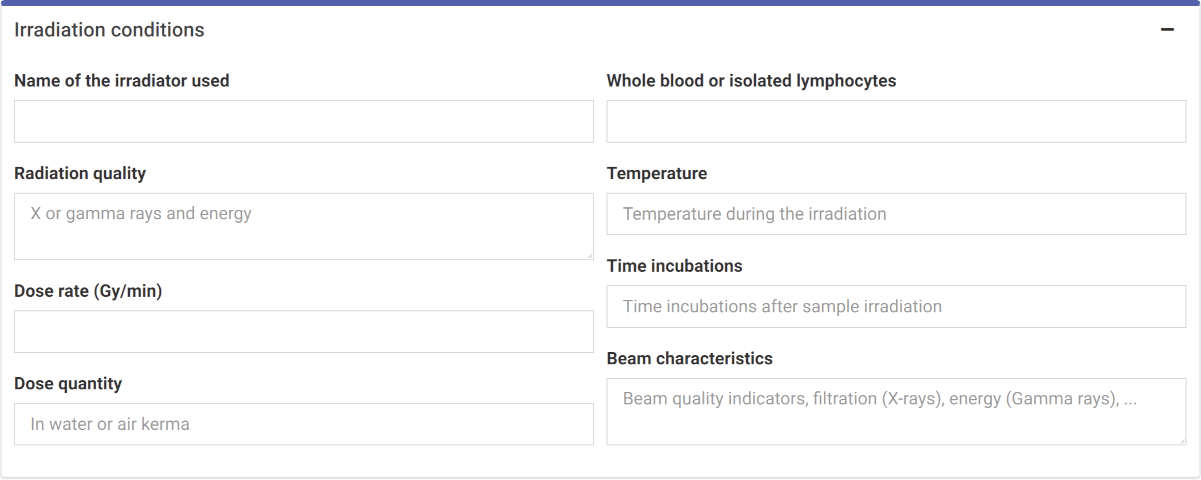‘Irradiation conditions’ box in the dose-effect fitting module

## Perform fitting

To perform the fitting the user needs select the appropriate fitting options to click the “Calculate fitting” button on the “Data input” box.

The fitting results and summary statistics are shown in the “Results” tabbed box, and the dose-effect curve is displayed in the “Curve plot” box.

The “Export results” box shows two buttons: (a) “Save fitting data”, and (b) “Download report”. The “Save fitting data” will generate an .rds file that contains all information about the count data, irradiation conditions, and options selected when performing the fitting. This file can be then loaded in the dose estimation module to load the dose-effect curve coefficients.

Similarly, the “Download report” will generate a .pdf or a .docx report containing all inputs and fitting results.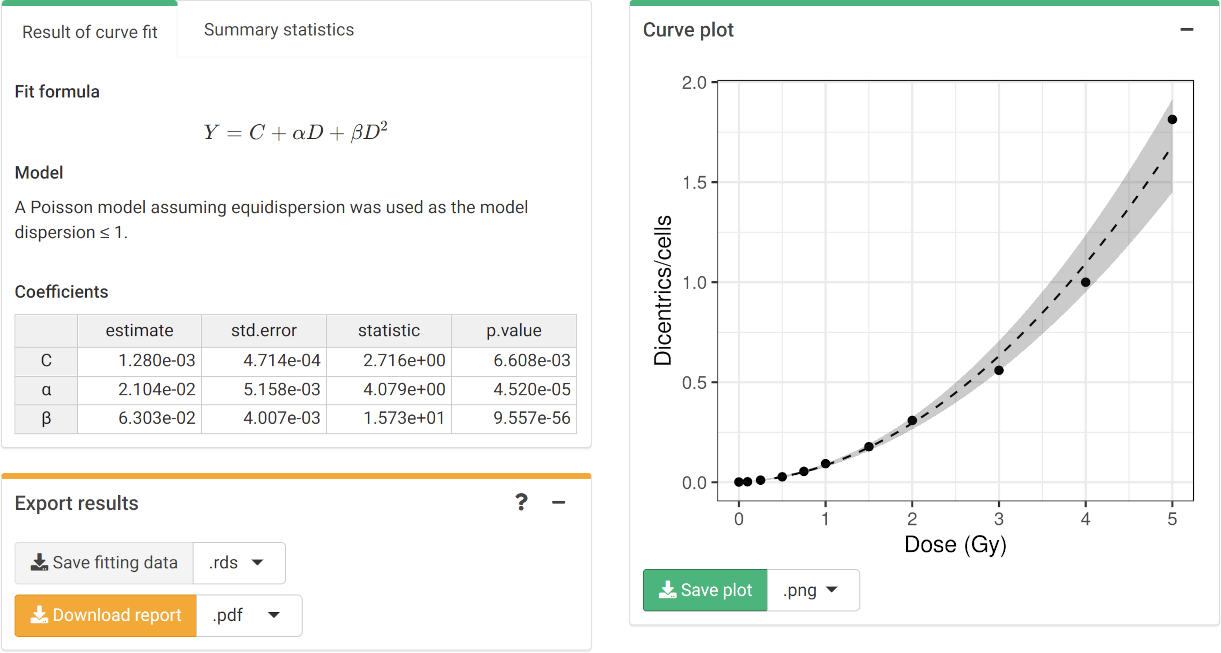‘Results’ tabbed box, ‘Curve plot’ and ‘Export results’ boxes in the dose-effect fitting module

To perform the fitting in R we call the fit() function:

fit_results <- fit(
count_data = count_data,
model_family = "automatic",
aberr_module = "dicentrics"
)

The fit_results object is a list that contains all necessary information about the count data as well as options selected when performing the fitting. This is a vital step to ensure traceability and reproducibility. Below we can see its elements:

names(fit_results)
#>   "fit_raw_data"         "fit_formula_raw"      "fit_formula_tex"
#>   "fit_coeffs"           "fit_cor_mat"          "fit_var_cov_mat"
#>   "fit_dispersion"       "fit_model_statistics" "fit_algorithm"
#>  "fit_model_summary"

In particular, we can see how fit_coeffs matches the results obtained in the UI:

fit_results\$fit_coeffs
#>                estimate    std.error statistic      p.value
#> coeff_C     0.001280319 0.0004714055  2.715961 6.608367e-03
#> coeff_alpha 0.021038724 0.0051576170  4.079156 4.519949e-05
#> coeff_beta  0.063032534 0.0040073856 15.729091 9.557291e-56

To visualise the dose-effect curve, we call the plot_fit_dose_curve() function:

plot_fit_dose_curve(
fit_results,
aberr_name = "Dicentrics"
)